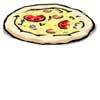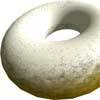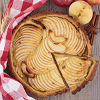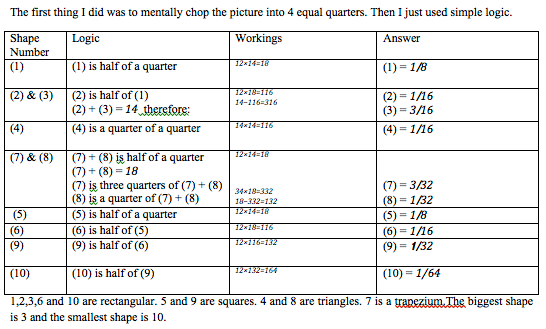#### You may also like### Pizza Portions

My friends and I love pizza. Can you help us share these pizzas equally?### Doughnut

How can you cut a doughnut into 8 equal pieces with only three cuts of a knife?### Pies

Grandma found her pie balanced on the scale with two weights and a quarter of a pie. So how heavy was each pie?

# Rectangle Tangle

##### Age 7 to 11Challenge Level

We had a large number of solutions sent in for this Rectangle Tangle challenge. Here is Zach's from U.K. which he sent in in table form:We had many solutions from The Hayes Primary School. Here are two as a taster from Arya and Matin.  The full solutions that the school sent in are here.doc (or here.pdf) and may serve as a useful discussion point in considering all the different ways of showing their solutions.

To tackle this problem I decided to work sysematically. Therefore, I first started with number 1. I looked carefully and saw the rectangle was split in half and that number 1 was a quarter of the half. The sum (calculation) would be $\frac{1}{4}$ of $\frac{1}{2}$ which equals $\frac{1}{8}$.
Next, I looked at number 2.  Easily, I could see it was a half of 1. So the answer would be $\frac{1}{8}$ of $\frac{1}{2}$ which equals $\frac{1}{16}$.
After that, I saw that number 3 was three times the size of number 2. The calculation would be $\frac{1}{16} \times3$. The result of this would be $\frac{3}{16}$.
Number 4 was a bit tricky. I looked at the two small triangles next to it and saw that joining them together would make number 4. This means that number 4 was a half of number 1. $\frac{1}{8}$ of $\frac{1}{2}$ is equal to $\frac{1}{16}$.
Obviously, number 5 was a quarter of a half.  $\frac{1}{4}$ of $\frac{1}{2}$ is equal to $\frac{1}{8}$.
Number 6 was a half of number 5 so the sum (calculation) was $\frac{1}{8}$ of $\frac{1}{2}$.  This equals $\frac{1}{16}$.
Number 7 was $\frac{3}{4}$ of 1. The sum was $\frac{3}{4}$ of $\frac{1}{8}$. As a result, the answer was $\frac{3}{32}$.
Number 8 was $\frac{1}{8}$ - $\frac{3}{32}$ because $\frac{3}{32}$ plus 8 would equal 1. The answer was $\frac{1}{32}$.
Number 9 was $\frac{1}{32}$ because it was a quarter of 5.  $\frac{1}{8}$ of $\frac{1}{4}$ is equal to $\frac{1}{32}$.
Finally, number 10 was a half of number 9. The sum (calculation) was $\frac{1}{32}$ of $\frac{1}{2}$.
The last solution was $\frac{1}{64}$.
To make sure, I added up all the calculations (including the ones that weren't numbered) and reached an answer of 1.

We also had many solutions sent in from Brabyns School which you can look at here.doc (or here.pdf).
As contrast we had this solution sent in from Murali Ryvers Primary School:

To solve this question, you can use the algebraic way and that is how I am going to show it out.

Firstly, I will replace the sides with letters. Let's suppose the longer sides are each y and the shorter sides with x. After doing that, multiply x and y together, making the area of the shape: xy. This information will help a lot.
Starting with an easier part to solve, we can take shape 5. The bottom and top side of it is fourth of y because it only covers quarter of the side.
The side parts are half of x. Times them together would make an eighth of the area so that should be shape 5's part: an eighth.
Shape 6 is obviously half of shape 5 by the look of it so that should be a sixteenth of the area so that is your answer for that part. Same is to shape 9. This is half of shape 6 visually so that would be 1 out of 32.
The next part is shape 10. That is half of shape 9 so that would be a sixty fourth of the area. Already four parts are covered.
Now we can move on to shape 1. By looking, you can see it is a quarter of one half of the rectangle so that means the shape one is an eighth of the whole rectangle. The last shape I am going to show is shape 2. This one is straight forward because it is half of shape 1! So that means it is a sixteenth of the total shape.
I am going to stop as you can see,I am running out of pages. I hope you enjoyed my explanation on how to work out the fraction of 6 out of 10 parts.
Thank you!

A late one that came in was from Sophia at Thorpe House Langley Prep.  You can see her work here.jpg
Finally from Year 5/6 Maths Challenge Group at Lyneham Primary School in Australia, we were sent the following good explanation from their teacher.

The students used a variety of different methods and arguments to find and justify their answers. There were some differences of opinion and someheated debate. It took a lot of persuasive power to reach agreement. In the end they decided that these were the fractions represented by each
shape :

Shape no.1 :$\frac{1}{8}$ .  no.2 :$\frac{1}{16}$ . no.3 : $\frac{3}{16}$. no.4 : $\frac{1}{16}$.  no.5 $\frac{1}{8}$. no.6 : $\frac{2}{32}$ or $\frac{1}{16}$. no.7 : $\frac{3}{32}$.no.8 : $\frac{1}{32}$. no.9 : $\frac{2}{32}$. no.10 : $\frac{1}{64}$

The students commented that it was easier to find the answers than to write up solutions. Several students were able to write partial solutions, transcribed below.

Shape no.1
M said : The no.1 shape is obviously $\frac{1}{8}$ because if you look at half the shape you can see that splitting it into quarters leaving four 1's. Therefore, putting the halves together makes it $\frac{1}{8}$.
KR said : Shape no.1 is 1/8 because if you look at the whole rectangle no.1 is in$\frac{1}{4}$ so you just half $\frac{1}{4}$ = $\frac{1}{8}$

Shape no.2
M said : As we already know, the 1's are all 1/8. If you filled the whole box with 2's, it would fit 16 because they are half the size of a 1.
KR said : Shape no.2 is $\frac{1}{16}$ because its half of shape no.1

Shape no.3
M said : As we can see, two 1's take up the same amount of space as a 2 and a 3. The 2, we know, equals half a 1, so then three 2's go into a 3, and because a 2 is $\frac{1}{16}$ a 3 is $\frac{3}{16}$.
KR said : No.3 is $\frac{3}{16}$ because if you stack three of no.2 it will =$\frac{3}{16}$.

Shape no.4
M said : 4 = $\frac{1}{16}$ because if you flip up the shapes on either side it fits perfectly, therefore it is basically the 1 cut in half and that means its half the fraction.
KR said : No.4 is also $\frac{1}{16}$ because if you deform no.2 and squiez it in no.4 it will make that shape.
KH wrote up a complete solution for all shapes, and even checked at the end that the fractions added to 1. I have attached this as a pdf document.

Thanks for another argument-provoking maths problem!

Just before this closed as a live activity we received solutions from year 6 at Broom Leys Primary School in Coalville, they were Jack, Matthew, Abi, Zoe, Maddie, Tom, Isaac, Sam, JJ, Aswin and Adam. Their solutions can be viewed here.doc or here.pdf

Well a big thank you to over 120 of you that sent in solutions, I hope you like comparing those that are published here. I am sorry that we can't show all of them but we would fill up much too much space. Enjoy the next new challenges that are on the site.